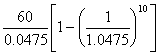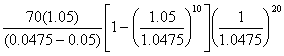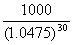Dr. P.V. Viswanath
 Home Bio Courses Research Economics/Finance on the Web Student InterestCoursesExams

Notes:

1. If your answers are not legible or are otherwise difficult to follow, I reserve the right not to give you any points.
2. If you cheat in any way, I reserve the right to give you no points for the exam, and to give you a failing grade for the course.
3. You may bring in sheets with formulas, but no worked-out examples, or definitions, or anything else.
5. Questions 6 and 7 are compulsory. Of the remaining questions, do any three.
6. Assume a tax rate of 40% where not provided.

Midterm

1. (20 points) According to the June 30, 2004 balance sheet of Sun Microsystems, it has a net working capital of \$2.19b, long-term assets of \$7.2b, long-term liabilities (total liabilities less current liabilities) of \$2.952b, and stockholders' equity of \$6.438b.

1. Create a balance sheet with net working capital and long-term assets shown on the assets side, and long-term liabilities and stockholders' equity shown on the liabilities side. Assume that all long-term liabilities represent long-term debt, whose service payments are tax-deductible. Now answer the following question: If the equity beta of SUNW is 2.772, what is your estimate of the firm beta?
2. If SUNW issued \$1b. of equity and bought back an equal amount of debt, what would its equity beta be?

2. (20 points) Altria (http://finance.yahoo.com/q/ks?s=MO) has an equity beta of 0.289. You estimate the market risk premium to be 6% per annum. If the yield on the 10-year T-bond is 4.22% (http://www.bloomberg.com), should Altria invest in a project that will have after-tax cashflows to equity of \$20b. a year for the first 5 years, and then increasing at the rate of 2% per annum forever? This project will require an initial investment of \$500b.

3. (20 points) You have been asked to value a 30-year, \$1000 face value bond, issued by CVS with the following features. The coupon rate for the first 10 years will be 6%of the face value. The coupon rate for the second 10 years will be 7% of face value. Thereafter, the coupon payment will increase at the rate of 5% per annum (coupon growth will be based on the dollar coupon the previous year). Write out the time line of cashflows, and estimate the value of this bond, if CVS is rated AA. (AA-rated bonds are trading at a default spread of 0.25% over the 10-year treasury bond rate of 4.50%.)

4. (20 points) You have data for the returns on Time Warner for the years 1999, 2000, 2001 and 2002. They are 95%, -54%, -8% and -59%. For the same period, the returns on the S&P 500 were 20%, -10%, -13% and -23%.

1. Compute the covariance between the returns on Time Warner and the returns on the S&P 500 portfolio.
2. Without computing, say whether Time Warner's beta would be greater or less than one and explain why. (No points without explanation.)

5. (20 points) In the November 4, 2004 edition of The Economist, an article titled, "Family businesses: Passing on the crown" had the following paragraph:

Because family-owned businesses have a more restricted access to capital than publicly quoted firms, they tend to concentrate in businesses that require relatively few assets, such as trading, consumer goods and retailing. There are exceptions: Vittorio Merloni has built his company into one of Europe's biggest manufacturers of white goods. And family firms are often more diversified than quoted companies—they are, after all, by far the family's main asset, and so the firm's own diversification makes family owners feel more secure. Shareholders in quoted companies have more scope to diversify their own portfolios by buying shares in other firms.

If, indeed, family businesses are more diversified than quoted companies, should they sell for more or less than quoted companies, assuming all other things are comparable?

6. (10 points) A bank can either lend to a corporate customer at an APR of 12% p.a., where the coupon payment schedule is semi-annual, or it can lend to another party, which will make equal monthly payments over a period of 10 years. What is the minimum rate that the bank should quote to this second party, if it does not want to make any less money on the deal than if it lent to its corporate customer?

7. (30 points) Answer any five of the following questions:

1. Why is a 3-month T-bill not riskless for somebody with a five-year horizon?
2. The beta for General Motors, estimated from a regression of stock returns against the S&P 500 index, is 1.2. If the standard error of the estimate is 0.30, can you reject the hypothesis that the true underlying beta is really unity?
3. Assuming the CAPM holds, is this statement true: If the variance of the overall market goes up, the betas of all firms will go down?
4. What is the required rate of return on a lottery ticket according to the CAPM? Explain.
5. What should be the required rate of return on the unlevered stock of a company that sells automobile insurance policies to drivers all over the country?
6. Can the book value of equity be negative? Why or why not?
7. You are comparing the profitability of two retail firms. One leases its stores using operating leases, and the other owns its stores. Which firm will show the higher operating income? Which one is likely to have a higher return on capital?
8. If the operating leases in the previous question were cancelable, which firm would have the higher beta?

Midterm Solutions

1. a.

 Assets Liabilities Net Working Capital 2.19b Long-term Liabilities 2.952b Long Term Assets 7.2b Stockholders' equity 6.438b Total 9.39b Total 9.39b

According to our assumptions, the relevant debt/equity ratio is 2.952/6.438 = 0.4585

The formula is: Unlevered/firm beta = levered beta/[1+(1-tax rate(D/E))] = 2.772/[1+(1-0.4)(0.4585)] = 2.1739

b. If SUNW issued \$1b of equity and bought back an equal amount of debt, its new D/E ratio would be 1.952/7.438 = 0.2624, and its equity beta would be 2.1739*[1+(1-0.4)(0.2624))] = 2.5162

2. The required rate of return on Altria is 4.22 + 0.289(6) = 5.954. Using this discount rate, the present value of the project can be computed as the sum of the present values of the annuity for the first five years plus the growing perpetuity, i.e. (20/0.0595)[1-(1.0595)-5] + 20(1.02)/[(0.0595-2)(1.0595)-5] = 84.36 + 386.84 = \$471.20. Since the initial required investment is \$500b, the project should not be undertaken.

3. 2. The PV of the cash flows for the first 10 years can be computed simply as.  The cash flows for the following 10 years have a present value equal to.  The final ten years have cashflows growing at the rate of 5% per annum; the present value of the cashflows during those ten years equals.  To this, we need to add the present value of the repayment of the principal, which is.  Adding all these values, together, we get 468.98 + 344 + 280.3636 + 248.53 = 1341.87

4.a.

 TMW Returns Sq. Dev from Mean S&P Returns Sq. Dev from Mean Product of deviations 1999 95 10302.25 20.00 702.25 2689.75 2000 -54 2256.25 -10.00 12.25 166.25 2001 -8 2.25 -13.00 42.25 9.75 2002 -59 2756.25 -23 272.25 866.25 Mean -6.5 5105.6667 -6.5 343 1244

The covariance of returns can be computed as the sum of the product of deviations, i.e. (2689.75 + 166.25 + 9.75 + 866.25), divided by the number of observations less one. This gives us the covariance as 1244.

b. Time Warner's beta should be greater than one. This can be seen by the fact that its stock returns have a greater amplitude than that of the S&P 500, which can be thought of as the market. (In our case, we can compute the beta as 1244/343 or 3.63, which is greater than one, as we expected.

5. Saying that family businesses should sell for more is the same as saying that quoted companies should sell for less because they are not as diversified. But individual investors can diversify their own portfolios for very little money by buying mutual funds or by otherwise using intermediaries. Hence there is no reason to believe that quoted companies are penalized for not being diversified.

6. If the coupon payment schedule is semi-annual and the APR is 12%, then the effective rate per six months is 6%. The effective annual rate, therefore, is (1.06)2-1 = 12.36%. If this were to be used as the effective annual rate for a loan with monthly repayments, the effective monthly rate on that loan would have to be (1.1236)1/12-1 = 0.009759 or .9759% or an APR of 0.9759x12 = 11.71%

7.a. A 3-month T-bill is not riskless for somebody with a five-year horizon because that investor would have to reinvest the money after 3 months, and even though the initial 3-month return is known, the return for the succeeding three month periods are uncertain; hence the investment itself is effectively not riskless.
b. If the returns are distributed normally, the 95% confidence intervals would be about 1.2 - 2(0.3), 1.2 + 2(0.3), which includes 1. Hence, one could not reject the hypothesis that the true underlying beta is really unity.
c. False. This is because the average beta is one. If the denominator rises, the relative size of the betas will not change,and hence, given the normalization to one, neither will the absolute values of the betas. In any case, all can never move in the same direction, since the average beta is one.
d. The required rate of return will be the riskfree rate because none of the uncertainty in a lottery ticket return is systematic.
e. Again, these automobile policies, being spread out over the country, the company's assets will be diversified. So, in this case, neither will the individual policies have non-zero betas, neither will the return on the stock itself be very uncertain. This assumes that accidents happen randomly, which may or may not be true. This also assumes that the company's investment strategy is given and does not vary with respect to the state of the economy.
f. The book value of equity can be negative because assets are usuallly valued on the balance sheet at cost; this can understate their true value. If the understatement is not so strong for the liabilities, book equity will end up having a negative value.
g. The firm which is leasing will end up deducting all its leasing costs as operating costs, even though the lease outlay really includes depreciation, as well. Hence it will have a lower operating income. However, it will also have a lower level of assets, since the assets generating the cashflows are not owned by the firm and don't show up in the balance sheet. As a result, it will probably have a higher return on capital.
h. If the operating leases were cancelable, then the firm with the leases would have more flexibility and hence the beta would be lower, since it would be more insulated against market downturns.